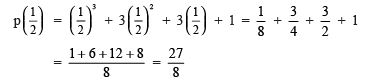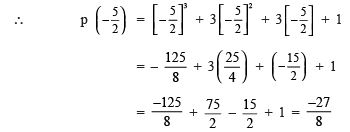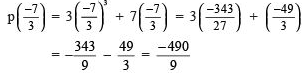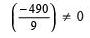Courses

# Ex 2.3 NCERT Solutions - Polynomials Class 9 Notes | EduRev

## Class 9 Mathematics by Full Circle

Created by: Full Circle

## Class 9 : Ex 2.3 NCERT Solutions - Polynomials Class 9 Notes | EduRev

The document Ex 2.3 NCERT Solutions - Polynomials Class 9 Notes | EduRev is a part of the Class 9 Course Class 9 Mathematics by Full Circle.
All you need of Class 9 at this link: Class 9

REMAINDER THEOREM

We know that: Dividend = (Divisor x Quotient) + Remainder

If in general, we denote:

Dividend as p(x),
Divisor as g(x),
Quotient as q(x),
Remainder as r(x).

Such that: (i) p(x), g(x), q(x) and r(x) are polynomials, and

(ii) g(x) ≠ 0

(iii) Degree of r(x) < Degree of g(x)

(iv ) Degree of g(x) ≤ Degree of p(x)

Then, we have p(x) = [g(x) . q(x)] + r(x)

Note: Theorem means a statement which is already proved.

Theorem: Let p(x) be any polynomial of degree greater than or equal to one and let ‘a’ be any real number.

If p(x) is divided by the linear polynomial (x – a), then the remainder is p(a).

Proof: Let p(x) be divided by (x – a) such that:

Quotient = q(x), Remainder = r(x)

∴ p(x) = [(x – a) . q(x)] + r(x) …(1)

Since, remainder is always smaller than the divisor.

∵ Divisor (x – a) is having degree as 1.

∴ Degree of remainder must be zero.

We know that the degree of a constant polynomial is zero.

∴ r(x) is a constant, say ‘r’.

Now, from (1), we have p(x) = [(x – a) . q(x)] + r

If x = a then P(a) = [(a – a) . q(a)] + r

or p(a) = [0 x (q (a))] + r

or p(a) = 0 + r

or p(a) = r i.e. Remainder = p(a).

Ques 1: Find the remainder when x3 + 3x2 + 3x + 1 is divided by (i) x + 1 (ii) x – 1/2(iii) x (iv) x + p ( v ) 5 + 2x

Ans: (i) ∵ The zero of x + 1 is –1 [∵ x + 1 = 0 ⇒ x = –1]

And by remainder theorem, when p(x) = x3 + 3x2 + 3x + 1 is divided by x + 1, then remainder is p(–1).

∴ p(–1) = (–1)3 + 3 (–1)2 + 3(–1) + 1 = –1 + (3 x 1) + (–3) + 1
= –1 + 3 – 3 + 1
= 0
Thus, the required remainder = 0

(ii) ∵ The zero of x –1/2 is 1/2     [∵ x –1/2 = 0 ﬁ x = 1/2]

and p(x) = x3 + 3x2 + 3x + 1

∴ For divisor = x – 1/2, remainder is given asThus, the required remainder = 27/8
(iii) We have p(x) = x3 + 3x2 + 3x + 1 and the zero of x is 0

∴ p(0) = (0)3 + 3(0)2 + 3(0) + 1 = 0 + 0 + 0 + 1 = 1

Thus, the required remainder = 1.

(iv) We have p(x) = x3 + 3x2 + 3x + 1 and zero of x + p = (–π)         [∵ x + π = 0 ⇒ x = – π]

∴ p(–π)= (– 5)3 + 3

(–π)2 + 3(–π) + 1 . = –π3 + 3(π2) + (–3π) + 1 = –p3 + 3π2 – 3π + 1

Thus, the required remainder is –π + 3π2 – 3π + 1.

(v) We have (p(x) = x+ 3x2 + 3x + 1 and zero of 5 + 2x is  5/2        [∵ 5 + 2x = 0 ⇒ x = -5/2]Thus, the required remainder is 27/8

Ques 2: Find the remainder when x3 – ax+ 6x – a is divided by x – a.
Ans:
We have p(x) = x3 – ax+ 6x – a

∵ Zero of x – a is a.      [∵ x – a = 0 ⇒ x = a]

∴ p(a) = (a)3 – a(a)2 + 6(a) – a = a3 – a3 + 6a – a = 0 + 5a = 5a

Thus, the required remainder = 5a

Ques 3: Check whether 7 + 3x is a factor of 3x3 + 7x.
Ans:
We have p(x) = 3x3 + 7x and zero of 7 + 3x is  -7/3       [∵ 7 + 3x = 0 ⇒ x = -7/3]sincei.e. the remainder is not 0.

∴ 3x– 7x is not divisible by 7 + 3x.

Thus, (7 + 3x) is not a factor of 3x3 – 7x.

132 docs

,

,

,

,

,

,

,

,

,

,

,

,

,

,

,

,

,

,

,

,

,

;